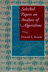# Selected Papers on Analysis of Algorithms

by Donald E. Knuth (Stanford, California: Center for the Study of Language and Information, 2000), xvi+622pp.

Here is a list of all significant changes that were made between the printing of 2008 and the printing of 2012. An asterisk (*) marks technical errors that are not merely typographical:

page 7, line 8 from the bottom
change '$\overline y_{ij}$' to '$y_{ij}$'
page 7, line 5 from the bottom
change '$\overline a$' to '$a$'
page 8, lines 5 and 6
change '$B$' to '$\overline B$', '$C$' to '$\overline C$', '$D$' to '$\overline D$', '$E$' to '$\overline E$', and '$F$' to '$\overline F$'
page 8, line 2 from the bottom
change '$A$' to '$\overline A$'
*page 16, lines 16 and 17
change '$k\ne l$' to '$l\ne j$' and '$k=l$' to '$l=j$'
page 30, line 4
change 'the set of all' to 'the sum of all'
page 31, line 6 from the bottom
change '$K_{t-s-3}(A_{s+4},\ldots,A_t)$' to $K_{t-s-3}(A_{s+4},\ldots,A_t)D$'
page 49, line 6
change 'some vertex' to 'some arc'
page 73, line 13
change 'Echecs Feeriques' to 'Échecs féeriques'
page 106, lines 24 and 25
change 'the best'' move' to 'a best'' move' and 'that the best' to 'that a best'
*page 134, in (42)
change '$1\le k\le$' to '$1\le k<$' (twice)
*page 155, bottom line
change '$\sum_{j=0}^{r-1}$' to '$\sum_{j=0}^r$'
*page 156, line 5
change '$r<s$' to '$s<r$'
page 161, line 16 from bottom
change '$C_3\ge$' to '$C_3\le$'
*page 163, line 1
change 'If $A_j=2$ for any $j$' to 'If $A_{j-1}=2$ for some $j$
*page 173, line 8
change '$+m_{j-1}$' to '$+m_{j+1}$' and '$+m_{j+2}$' to '$-m_{j+2}$'
page 227, line 20
change "idea " to "idea (see  and )"
page 233, replacement for reference 
 J. Aebly, Démonstration du problème du scrutin par des considerations geometriques,'' L'Enseignement Mathématique 23 (1923), 185--186.
page 233, move reference  to  and insert a new reference 
 D. Mirimanoff, A propos de l'interpretation geometrique du problème du scrutin,'' L'Enseignement Mathématique 23 (1923), 187--189.
*page 237, line 11 from the bottom
change '$\rho_2=(0\ 3\ 2)$' to '$\rho_2=(0\ 1\ 3)$'
*page 238, lines 15 and 17
change '$m_t$' to '$m_{t-1}$' (four changes)
*page 244, line 2 from the bottom
change '$d^{h-k+1}$' to '$d^{h-1+k}$'
page 246, line 8 from the bottom
change '$P_{hd}(k)$' to '$p_{hd}(k)$'
page 272, line 4 from the bottom
change '$dt$' to '$dt\,dx\,dy$' (two changes)
page 286, line 14
change '$y'_{n-1+m}$' to '$y'_{n-1-m}$'
page 293, line 17
change '$I^*DI_o^*$' to '$I_o^*DI_o^*$'
*page 310, line 12
change '$\int_1^{x^{\alpha-1}}$' to '$x^\alpha \int_1^{x^{\alpha-1}}$'
*page 310, line 6 from the bottom
change '$1\over n-1$' to '$1\over n+1$'
*page 311, line 2 from the bottom
change '$x^\alpha\int_1^2$' to '$\int_1^2$'
*page 312, lines 7 and 8
change '$x^{2/\alpha}\,dt$' to $x^{2\alpha/t}\,dt\over\alpha$' and '$x^{\alpha u}\,du\over u^2$' to '$x^{\alpha u}\,du\over 2\alpha u^2$'
page 316, line 4 from the bottom
change '$\rho(t)$' to '$\rho_k(t)$'
page 344, line 1
change '$e^t$' to '$e^{-t}$'
*page 347, bottom line
change '$\int_0^1\exp(cn^{3/2}t)$' to '$\int_0^t\exp(cn^{3/2}u)$'
*page 351, line 2 from the bottom
change '$\exp$' to '$t^{k+m-2}\exp$'
*page 358, bottom line
change '${1\over n-c\sqrt k-1}\ldots{1\over n-c\sqrt k-k}$' to '${1\over n-c\sqrt k-1}+\cdots+{1\over n-c\sqrt k-k}$'
page 398, line 11 from the bottom
change 'using André's well-' to 'using a well-'
page 399, line 3 from the bottom
change 'by André's reflection' to 'by the reflection'
page 434, line 7
change '$l,p_1,h$' to '$l,p,h$'
page 457, line 5 from the bottom
change '$Q^*_{kl}$' to '$Q^*_l$'
page 464, line 7 from the bottom
change 'by (7.6)' to 'by (7.7)'
page 470, line 7
change '$2^{-n-1}b$' to '$2^{-n}b$'
page 472, new copy
Nicholas Pippenger [“Analysis of carry propagation in addition: An elementary approach,” Journal of Algorithms 42 (2002), 317--333] has explained how to obtain these results without using contour integration.
page 485, line 17 from the bottom
change 'Hamiltonian circuit' to 'Hamiltonian cycle'
page 500, replacement for the final paragraph
Marcin Peczarski [New results in minimum-comparison sorting,'' Algorithmica 40 (2004), 133--145; The Ford--Johnson algorithm still unbeaten for less than 47 elements,'' Information Processing Letters 101 (2007), 126--128] has extended Wells's method to prove that merge insertion is actually unbeatable when $n=13$, 14, or 15. The optimum procedure for sorting 16 elements remains unknown.
page 526, line 3 from the bottom
put an end-of-proof box after '$\le k$?'
page 528, in reference 
change 'SIAM Journal of Applied' to 'SIAM Journal on Applied'
page 537, line 14
change '$\bigvee_{n\in A_j}$' to '$\bigvee_{a\in A_j}$'
page 559, line 6 from the bottom
change 'If $n$ is odd$' to 'If$n>1$is odd' *page 563, lines 8 and 9 following (3.9) change '$0<\theta$' to '$0\le\theta$' and '$n>0$' to '$n\ge0$' page 568, line 9 change '$p+(1-p_1)$' to '$p_1+(1-p_1)$' page 571, line 19 change 'know from (1.6)' to 'know from (1.5)' page 578, line 8 change '$\varepsilon_m\bigl(f(x)\bigr)$' to '$\varepsilon_m\bigl(f(x)\bigr)\,dx$' page 591, in (7.6) change '$(1-t)^b$' to '$(1-t)^b\,dt$' page 604, new paragraph Philippe Flajolet, Maryse Pelletier, and Michèle Soria [On Buffon machines and numbers,'' ACM--SIAM Symposium on Discrete Algorithms 22 (2011), 172--183] have substantially generalized many of the topics treated in this chapter. In particular, they have analyzed the process of generating the Poisson distribution with an arbitrary parameter$\lambda\ge0\$.
page 605, right column
change "André, Antoine Désiré, 233, 398--399" to "Aebly, Jakob, 233" (and re-alphabetize)
page 607, left column, new entry
Buffon, Georges Louis Leclerc, Comte de, 604.
page 607, right column
change 'Chvátal, Václav' to 'Chvátal, Václav (= Vašek)'
page 610, left column, Flajolet entry
page 614, left column
matchbox problem, 231--234.
page 614, right column, new entry
Mirimanoff, Dimitry, 233.
page 616, left column, new entry
Pelletier, Maryse, 604.
page 616, right column, Pippenger entry###Don Knuth's other books